Image Processing Toolbox User's GuideDiscrete Cosine Transform

The discrete cosine transform (DCT) represents an image as a sum of sinusoids of varying magnitudes and frequencies. The `dct2` function in the Image Processing Toolbox computes the two-dimensional discrete cosine transform (DCT) of an image. The DCT has the property that, for a typical image, most of the visually significant information about the image is concentrated in just a few coefficients of the DCT. For this reason, the DCT is often used in image compression applications. For example, the DCT is at the heart of the international standard lossy image compression algorithm known as JPEG. (The name comes from the working group that developed the standard: the Joint Photographic Experts Group.)

The two-dimensional DCT of an M-by-N matrix `A` is defined as follows.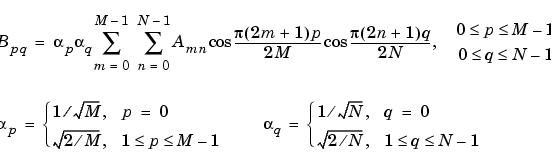The values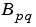are called the DCT coefficients of `A`. (Note that matrix indices in MATLAB always start at 1 rather than 0; therefore, the MATLAB matrix elements `A(1,1)` and `B(1,1)` correspond to the mathematical quantities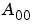and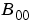, respectively.)

The DCT is an invertible transform, and its inverse is given by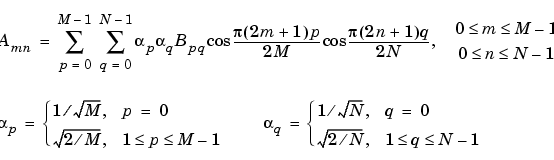The inverse DCT equation can be interpreted as meaning that any M-by-N matrix `A` can be written as a sum of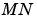functions of the form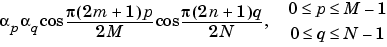These functions are called the basis functions of the DCT. The DCT coefficients, then, can be regarded as the weights applied to each basis function. For 8-by-8 matrices, the 64 basis functions are illustrated by this image.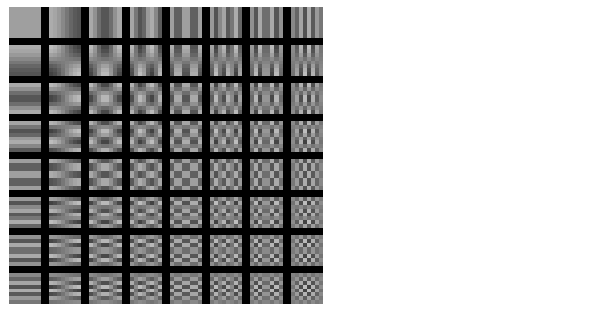The 64 Basis Functions of an 8-by-8 Matrix

Horizontal frequencies increase from left to right, and vertical frequencies increase from top to bottom. The constant-valued basis function at the upper left is often called the DC basis function, and the corresponding DCT coefficientis often called the DC coefficient.Applications of the Fourier Transform The DCT Transform Matrix